Home > Mini Projects > ASK Modulation using OPAMP

Abstract: Amplitude Shift Keying plays a major role in the field of digital modulation because of its simplicity in implementation as well as detection. ASK involves multiplier circuit with data stream and a high frequency carrier signal as input. The resulting ASK modulated output signal is highly band efficient.

I. INTRODUCTION1

In the world of electronics and telecommunications, modulation implies variation in some parameter of the carrier signal by means of the signal that is to be transmitted. In earlier days, communication was mostly achieved using analog domain .However, there were many drawbacks in this case. With the advent advancement in research, digital domain was proven to be far more efficient over analog.

The baseband signal cannot be transmitted over long distances because of its low frequency. Consequently, this baseband signal has to be modulated in order to shift its frequency to RF range. Among various available modulation techniques, ASK is the simplest modulation technique being discussed here.

II. CIRCUIT IMPLEMENTATION A. Operational Amplifier

An Operational Amplifier, or op-amp for short is basically a voltage amplifying device with 2 terminals as input and one terminal as output. It requires external feedback to control its overall response characteristics or operation. Differential amplifier is the basic building block of op-amp. The differential amplifier stage is followed by a level translator and then an output stage.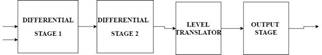Op-amp has infinite input impedence resulting in no current flowing in either of its input terminals and zero input offset voltage. Further it has zero output resistance. It is a three terminal device having two input terminals: Inverting terminal (-) and Non-Inverting terminal (+) and anoutput terminal. The difference of the voltages applied at input terminals is amplified by gain value. It can work as an ideal amplifier with infinite gain and bandwidth when used in the open -loop mode.

B. Logarithmic Amplifier

An operational amplifier can function as a logarithmic amplifier in which output of the op-amp is log of the input. It is said to be configured in non-linear mode. In this circuit, a diode is placed in the feedback path of op-amp. When the diode is forward biased by a constant current of magnitude Vi/R, it develops a potential:Where Vd is potential across diode

Vt is thermal voltage

R is resistance at inverting terminal

Io is saturation current

Vi is input voltage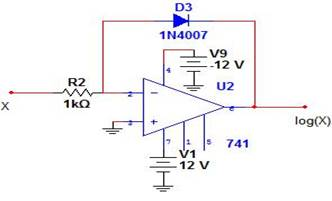C. Anti-Logarithmic Amplifier

An operational amplifier can function as an anti-logarithmic amplifier in which output of the op-amp is anti-log of the input. It is said to be configured in non-linear mode. In this circuit, a diode is placed at the inverting terminal input and a diode in the feedback network. When the diode is forward biased by a constant current of magnitude Vi/R, it develops a potential: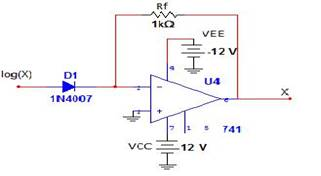D. Summing Amplifier

The op-amp amplifies each input voltage by gain value and produces an algebraic sum of these inputs at the output terminal. In case Inverting Summing Amplifier, the output is

Vout = - ( )Where v1, v2, v3 are three input voltages to be summed

up

Rf is feedback resistance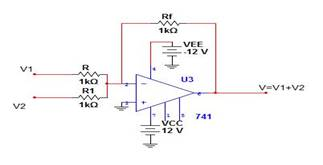E. Multiplier Circuit

Here the multiplier circuit is implemented using Log, anti-log and summing amplifier circuits discussed previously.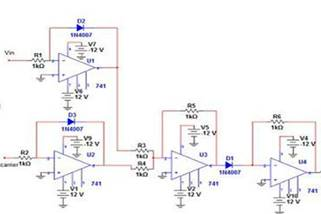The ASK modulator is implemented using the multiplier circuit discussed above. To one of the log amplifiers data stream is given as input and to the other the high frequency carrier is given as input. The output of multiplier circuit is given to an inverting amplifier as in the multiplier stage op-amp is used in inverting configuration.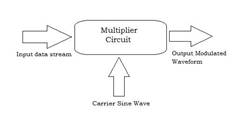CIRCUIT DIAGRAM: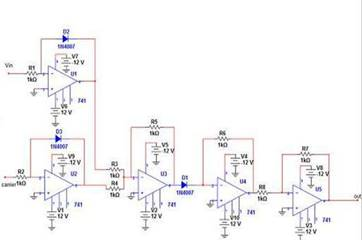Op-amp subcircuit:

.subckt opamp 1 2 6

E1 3 0 2 1 100k

E2 5 0 4 0 1

R1 1 2 100meg

r2 3 4 1k

r3 5 6 10k

c1 4 0 1.6u

.ends opamp

*mod

.include op.cir

v1 1 0 sin(0 10 20k)

v2 2 0 pulse(0 10 0 0 0 1ms 2ms)

r1 1 3 1k

r2 2 4 1k

x1 3 0 5 opamp

x2 4 0 6 opamp

d2 3 5

d3 4 6

r3 5 7 1k

r4 6 7 1k

x3 7 0 8 opamp

r5 7 8 1k

d1 8 10

x4 10 0 11 opamp

r6 10 11 1k

x5 12 0 13 opamp

r7 11 12 1k

r8 12 13 10k

.tran 1u 10ms

.control

run

display

set color0=white

set color1=black

set xbrushwidth=3

plot v(1)

plot v(2)

plot v(13)

.endc

.end

Input Waveforms:

a) Carrier wave: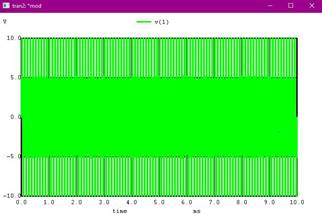b) Data to be modulated: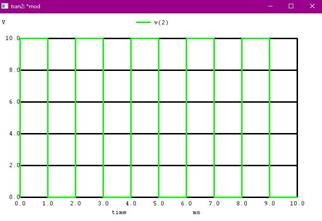3

Output: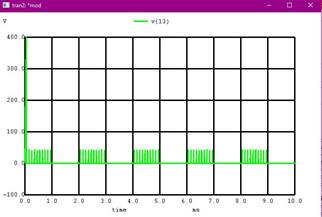III. CONCLUSION

ASK modulation has been implemented using NGSPICE simulator. The multiplier circuit implemented using op-amp Generated the desired results in accordance to the input data stream and carrier.

ASK is widely accepted due to its simplicity in implementation as well as detection. It requires lesser bandwidth when compared to FSK.

However it is more prone to noise leading to usage only in more noise immune channels like Optical fibres.